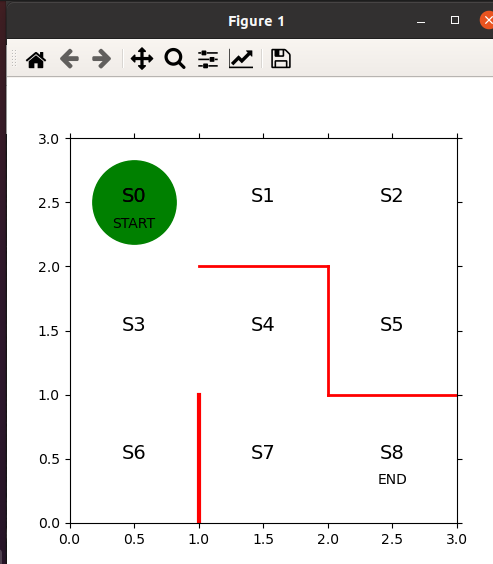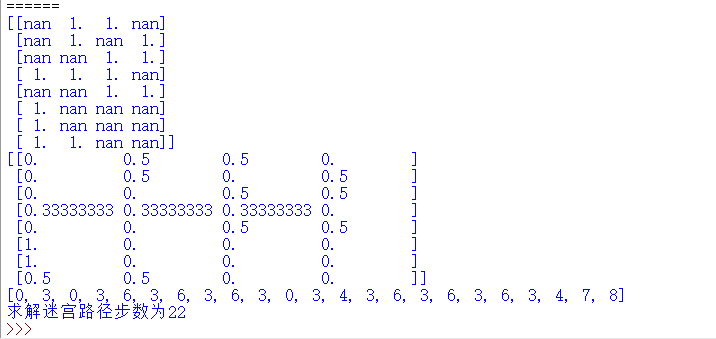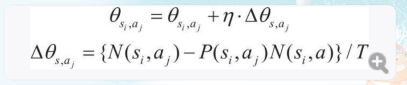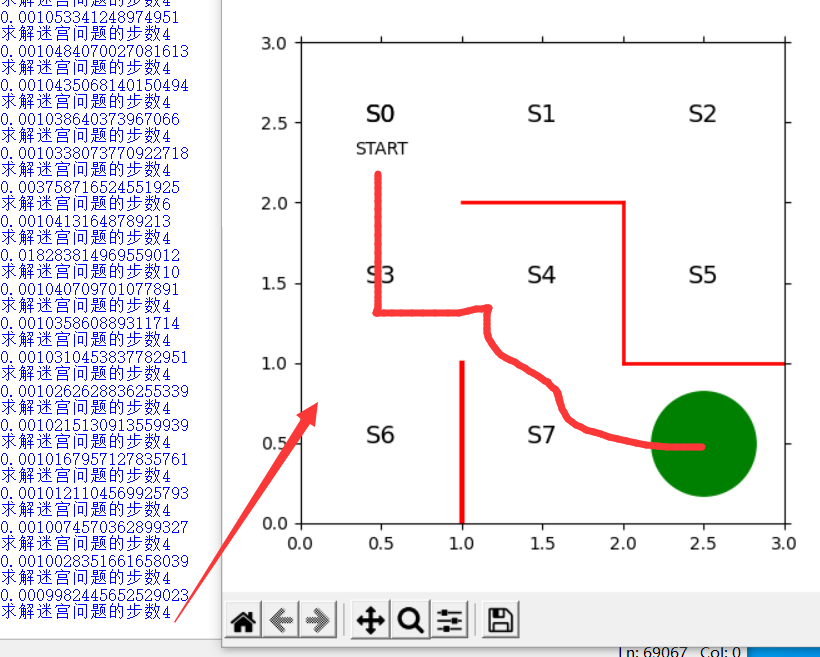<sub id="gqw76"><listing id="gqw76"></listing></sub>
<sub id="gqw76"><listing id="gqw76"></listing></sub>

1. <form id="gqw76"><legend id="gqw76"></legend></form>

# 0x00 機器學習基礎

機器學習可分為三類

• 監督學習
• 無監督學習
• 強化學習

三種學習類別的關鍵點

• 監督學習需要人為設置參數，設置好標簽，然后將數據集分配到不同標簽。
• 無監督學習同樣需要設定參數，對無標簽的數據集進行分組。
• 強化學習需要人為設置初始參數，然后通過數據的反饋，不斷修改參數，使得函數出現最優解，也即我們認為最完美的策略。

機器學習的原理

• 向系統提供數據（訓練數據或者學習數據） 并通過數據自動確定系統的參數。

機器學習常見算法有很多，比如

• 邏輯回歸
• 支持向量機
• 決策樹
• 隨機森林
• 神經網絡

但是是否真的需要按照概率論->線性代數->高等數學->機器學習->深度學習->神經網絡這個順序去學呢？

• 不一定，因為等你學完，可能會發現自己真正需要的一些東西學的不夠清楚，反倒是學了一堆只是看過一次之后就不會用到的知識點。

# 0x01 強化學習背景

??強化學習剛出現時非?；鸨?，但是之后卻逐漸變冷。主要原因在于強化學習不能很好的解決狀態縮減表示，智能體主要存在著狀態和動作，比如狀態可看作人類在地球的某個位置，動作可看作人類走路，如果我們很清楚人類的位置和動作其實就能預測出人的下一個狀態和動作，但是事實上智能體需要通過枚舉的方式將所有可能的狀態匯集到一張表中，而就我們這個例子來說，一個人的下一個狀態實在是太多了，比如現在我位于北京，我下一步飛到上海，飛到南京，沒有誰清楚我到底飛哪里。為什么AI不能突破到強人工智能？我認為主要還是算力不夠，沒辦法把智能體的所有狀態都列舉出來?，F今因為深度學習可以對大量的數據進行降維處理，使得數據集保有了特征的同時縮小了體積，使得同樣的算力情況下，強化學習能夠收集到智能體更多的狀態用來獲得最優解，所以出現了新的概念，叫做深度強化學習，它是將深度學習與強化學習結合的強化學習的加強版，這種學習可以完成對于人類來說非常困難的任務。

# 0x02 迷宮的建立

import numpy as np
import matplotlib.pyplot as plt

fig=plt.figure(figsize=(5,5))
ax=plt.gca()
# 畫墻壁
plt.plot([1,1],[0,1],color='red',linewidth=3)
plt.plot([1,2],[2,2],color='red',linewidth=2)
plt.plot([2,2],[2,1],color='red',linewidth=2)
plt.plot([2,3],[1,1],color='red',linewidth=2)
# 畫狀態
plt.text(0.5,2.5,'S0',size=14,ha='center')
plt.text(1.5,2.5,'S1',size=14,ha='center')
plt.text(2.5,2.5,'S2',size=14,ha='center')
plt.text(0.5,1.5,'S3',size=14,ha='center')
plt.text(1.5,1.5,'S4',size=14,ha='center')
plt.text(2.5,1.5,'S5',size=14,ha='center')
plt.text(0.5,0.5,'S6',size=14,ha='center')
plt.text(1.5,0.5,'S7',size=14,ha='center')
plt.text(2.5,0.5,'S8',size=14,ha='center')
plt.text(0.5,2.5,'S0',size=14,ha='center')
plt.text(0.5,2.3,'START',ha='center')
plt.text(2.5,0.3,'END',ha='center')
# 設置畫圖范圍
ax.set_xlim(0,3)
ax.set_ylim(0,3)
plt.tick_params(axis='both',which='both',bottom='off',top='off',labelbottom='off',right='off',left='off',labelleft='off')
# 當前位置S0用綠色圓圈
line,=ax.plot([0.5],[2.5],marker="o",color='g',markersize=60)
# 顯示圖
plt.show()


運行結果# 0x03 策略迭代算法

??對于我們人類而言，一眼就可以看出怎么從START走到END S0->S3->S4->S7-S8
那么對于機器而言，我們正常套路是通過編程直接寫好路線解決這種問題，不過這樣的程序依賴的主要是我們自己的想法了，現在我們要做的是強化學習，是讓機器自己根據數據學習怎么走路線。

基本概念

• 強化學習中定義智能體的行為方式的規則稱為策略 policy 策略 使用 Πθ(s,a)來表示 ，意思是在狀態s下采取動作a的概率遵循由參數θ決定的策略Π。

在這里，狀態指的是智能體在迷宮的位置，動作指的是向上、右、下、左的四種移動方式。
Π可用各種方式表達，有時是函數的形式。
這里可通過表格的方式，行表示狀態，列表示動作，對應的值表示概率來清楚的表示智能體下一步運動的概率。

若Π是函數，則θ是函數中的參數，在這里表格中，θ表示一個值，用來轉換在s狀態下采取a的概率。

定義初始值

theta_0=np.array([[np.nan,1,1,np.nan], #S0
[np.nan,1,np.nan,1], #S1
[np.nan,np.nan,1,1], #S2
[1,1,1,np.nan], #S3
[np.nan,np.nan,1,1], #S4
[1,np.nan,np.nan,np.nan], #S5
[1,np.nan,np.nan,np.nan], #S6
[1,1,np.nan,np.nan],  #S7
])   # S8位目標 不需要策略


將θ值轉成百分比

def int_convert_(theta):
[m,n]=theta.shape # 獲取矩陣大小
pi=np.zeros((m,n))
for i in range(0,m):
pi[i,:]=theta[i,:] / np.nansum(theta[i,:]) # 計算百分比
pi=np.nan_to_num(pi) # 將nan轉換成0
return pi


運行結果就目前來說，可滿足不撞墻，并且隨機移動的策略。

定義移動的狀態

def get_next_s(pi,s):
direction=["up","right","down","left"]
next_direction=np.random.choice(direction,p=pi[s,:])
# 根據概率去選擇方向
if next_direction=="up":
s_next=s-3 # 向上移動時狀態數字-3
if next_direction=="right":
s_next=s+1 # 向右移動時狀態數字+1
if next_direction=="down":
s_next=s+3 # 向下移動時狀態數字+3
if next_direction=="left":
s_next=s-1 # 向左移動時狀態數字-1
return s_next


定義最終的狀態

def goal_maze(pi): # 根據定義的策略持續移動.
s=0 # 設置開始地點
state_history=  # 記錄智能體軌跡的列表
while (1): # 循環執行，直到智能體到達終點
next_s=get_next_s(pi,s)
state_history.append(next_s) # 在記錄表中記錄下一步狀態
if next_s==8: # 到達終點
break
else:
s=next_s
return state_history


根據我們所設想的，最短路徑是下右下右 此時的狀態是8 所以選擇狀態為8 表示最終路徑。
但實際上智能體根據我們開始設置好的θ值去運動的話，是隨機運動，只要結果為8就停止，所以有各種各樣的運動路徑，我們需要做的是想辦法讓智能體自己學習走一條最短路徑

上訴代碼運行實例第一個結果為原θ 第二個結果為我們轉換成概率的θ，第三個結果為設置智能體的狀態，未設置動作，然后根據θ指定策略，通過該策略讓智能體持續移動，可能會產生各種各樣的結果
上述完整代碼

import numpy as np
import matplotlib.pyplot as plt
def plot():
fig=plt.figure(figsize=(5,5))
ax=plt.gca()
# 畫墻壁
plt.plot([1,1],[0,1],color='red',linewidth=3)
plt.plot([1,2],[2,2],color='red',linewidth=2)
plt.plot([2,2],[2,1],color='red',linewidth=2)
plt.plot([2,3],[1,1],color='red',linewidth=2)
# 畫狀態
plt.text(0.5,2.5,'S0',size=14,ha='center')
plt.text(1.5,2.5,'S1',size=14,ha='center')
plt.text(2.5,2.5,'S2',size=14,ha='center')
plt.text(0.5,1.5,'S3',size=14,ha='center')
plt.text(1.5,1.5,'S4',size=14,ha='center')
plt.text(2.5,1.5,'S5',size=14,ha='center')
plt.text(0.5,0.5,'S6',size=14,ha='center')
plt.text(1.5,0.5,'S7',size=14,ha='center')
plt.text(2.5,0.5,'S8',size=14,ha='center')
plt.text(0.5,2.5,'S0',size=14,ha='center')
plt.text(0.5,2.3,'START',ha='center')
plt.text(2.5,0.3,'END',ha='center')
# 設置畫圖范圍
ax.set_xlim(0,3)
ax.set_ylim(0,3)
plt.tick_params(axis='both',which='both',bottom='off',top='off',labelbottom='off',right='off',left='off',labelleft='off')
# 當前位置S0用綠色圓圈
line,=ax.plot([0.5],[2.5],marker="o",color='g',markersize=60)
# 顯示圖
plt.show()
def int_convert_(theta): # 設置策略中的參數θ
[m,n]=theta.shape # 獲取矩陣大小
pi=np.zeros((m,n))
# print(pi,m,n)
for i in range(0,m):
pi[i,:]=theta[i,:] / np.nansum(theta[i,:]) # 通過循環遍歷行，對每行里的值進行計算百分比
#nansum(theta[i,:]) 為除了nan之外的數字的個數和
pi=np.nan_to_num(pi) # 將nan轉換成0
return pi

def get_next_s(pi,s): # 設置智能體的下一步狀態
direction=["up","right","down","left"]
next_direction=np.random.choice(direction,p=pi[s,:])
# 根據概率去選擇方向
if next_direction=="up":
s_next=s-3 # 向上移動時狀態數字-3
if next_direction=="right":
s_next=s+1 # 向右移動時狀態數字+1
if next_direction=="down":
s_next=s+3 # 向下移動時狀態數字+3
if next_direction=="left":
s_next=s-1 # 向左移動時狀態數字-1
return s_next

def goal_maze(pi): # 根據定義的策略持續移動.
s=0 # 設置開始地點
state_history=  # 記錄智能體軌跡的列表
while (1): # 循環執行，直到智能體到達終點
next_s=get_next_s(pi,s)
state_history.append(next_s) # 在記錄表中記錄下一步狀態
if next_s==8: # 到達終點
break
else:
s=next_s
return state_history

if __name__=="__main__":
theta_0=np.array([[np.nan,1,1,np.nan], #S0
[np.nan,1,np.nan,1], #S1
[np.nan,np.nan,1,1], #S2
[1,1,1,np.nan], #S3
[np.nan,np.nan,1,1], #S4
[1,np.nan,np.nan,np.nan], #S5
[1,np.nan,np.nan,np.nan], #S6
[1,1,np.nan,np.nan],  #S7
])   # S8位目標 不需要策略
print(theta_0)
print(int_convert_(theta_0))
state_history=goal_maze(int_convert_(theta_0))
print(state_history)
print("求解迷宮路徑步數為"+str(len(state_history)-1))
plot()


查看智能體運動軌跡

import numpy as np
import matplotlib.pyplot as plt
from matplotlib import animation
from IPython.display import HTML
def plot():
fig=plt.figure(figsize=(5,5))
ax=plt.gca()
# 畫墻壁
plt.plot([1,1],[0,1],color='red',linewidth=3)
plt.plot([1,2],[2,2],color='red',linewidth=2)
plt.plot([2,2],[2,1],color='red',linewidth=2)
plt.plot([2,3],[1,1],color='red',linewidth=2)
# 畫狀態
plt.text(0.5,2.5,'S0',size=14,ha='center')
plt.text(1.5,2.5,'S1',size=14,ha='center')
plt.text(2.5,2.5,'S2',size=14,ha='center')
plt.text(0.5,1.5,'S3',size=14,ha='center')
plt.text(1.5,1.5,'S4',size=14,ha='center')
plt.text(2.5,1.5,'S5',size=14,ha='center')
plt.text(0.5,0.5,'S6',size=14,ha='center')
plt.text(1.5,0.5,'S7',size=14,ha='center')
plt.text(2.5,0.5,'S8',size=14,ha='center')
plt.text(0.5,2.5,'S0',size=14,ha='center')
plt.text(0.5,2.3,'START',ha='center')
plt.text(2.5,0.3,'END',ha='center')
# 設置畫圖范圍
ax.set_xlim(0,3)
ax.set_ylim(0,3)
plt.tick_params(axis='both',which='both',bottom='off',top='off',labelbottom='off',right='off',left='off',labelleft='off')
# 當前位置S0用綠色圓圈
line,=ax.plot([0.5],[2.5],marker="o",color='g',markersize=60)
# 顯示圖
plt.show()
def int_convert_(theta): # 設置策略中的參數θ
[m,n]=theta.shape # 獲取矩陣大小
pi=np.zeros((m,n))
# print(pi,m,n)
for i in range(0,m):
pi[i,:]=theta[i,:] / np.nansum(theta[i,:]) # 通過循環遍歷行，對每行里的值進行計算百分比
#nansum(theta[i,:]) 為除了nan之外的數字的個數和
pi=np.nan_to_num(pi) # 將nan轉換成0
return pi

def get_next_s(pi,s): # 設置智能體的下一步狀態
direction=["up","right","down","left"]
next_direction=np.random.choice(direction,p=pi[s,:])
# 根據概率去選擇方向
if next_direction=="up":
s_next=s-3 # 向上移動時狀態數字-3
if next_direction=="right":
s_next=s+1 # 向右移動時狀態數字+1
if next_direction=="down":
s_next=s+3 # 向下移動時狀態數字+3
if next_direction=="left":
s_next=s-1 # 向左移動時狀態數字-1
return s_next

def goal_maze(pi): # 根據定義的策略持續移動.
s=0 # 設置開始地點
state_history=  # 記錄智能體軌跡的列表
while (1): # 循環執行，直到智能體到達終點
next_s=get_next_s(pi,s)
state_history.append(next_s) # 在記錄表中記錄下一步狀態
if next_s==8: # 到達終點
break
else:
s=next_s
return state_history

# 動畫展示
def init():
# 初始化背景
line.set_data([],[])
return (line,)

def animate(i):
# 每一幀的畫面
state=state_history[i]
x=(state % 3)+0.5
y=2.5-int(state/3)
line.set_data(x,y)
return (line,)

if __name__=="__main__":
theta_0=np.array([[np.nan,1,1,np.nan], #S0
[np.nan,1,np.nan,1], #S1
[np.nan,np.nan,1,1], #S2
[1,1,1,np.nan], #S3
[np.nan,np.nan,1,1], #S4
[1,np.nan,np.nan,np.nan], #S5
[1,np.nan,np.nan,np.nan], #S6
[1,1,np.nan,np.nan],  #S7
])   # S8位目標 不需要策略
print(theta_0)
print(int_convert_(theta_0))
state_history=goal_maze(int_convert_(theta_0))
print(state_history)
print("求解迷宮路徑步數為"+str(len(state_history)-1))
fig=plt.figure(figsize=(5,5))
ax=plt.gca()
# 畫墻壁
plt.plot([1,1],[0,1],color='red',linewidth=3)
plt.plot([1,2],[2,2],color='red',linewidth=2)
plt.plot([2,2],[2,1],color='red',linewidth=2)
plt.plot([2,3],[1,1],color='red',linewidth=2)
# 畫狀態
plt.text(0.5,2.5,'S0',size=14,ha='center')
plt.text(1.5,2.5,'S1',size=14,ha='center')
plt.text(2.5,2.5,'S2',size=14,ha='center')
plt.text(0.5,1.5,'S3',size=14,ha='center')
plt.text(1.5,1.5,'S4',size=14,ha='center')
plt.text(2.5,1.5,'S5',size=14,ha='center')
plt.text(0.5,0.5,'S6',size=14,ha='center')
plt.text(1.5,0.5,'S7',size=14,ha='center')
plt.text(2.5,0.5,'S8',size=14,ha='center')
plt.text(0.5,2.5,'S0',size=14,ha='center')
plt.text(0.5,2.3,'START',ha='center')
plt.text(2.5,0.3,'END',ha='center')
# 設置畫圖范圍
ax.set_xlim(0,3)
ax.set_ylim(0,3)
plt.tick_params(axis='both',which='both',bottom='off',top='off',labelbottom='off',right='off',left='off',labelleft='off')
# 當前位置S0用綠色圓圈
line,=ax.plot([0.5],[2.5],marker="o",color='g',markersize=60)
anim=animation.FuncAnimation(fig,animate,init_func=init,frames=len(state_history),interval=20,repeat=False,blit=True)
plt.show()


看動畫很直觀的反映，智能體雖然最終會到S8，但是并不是真的直接到S8,我們需要通過強化學習算法來讓智能體學會怎么樣以最短路徑到達S8

主要有2種方式

• 根據策略到達目標時，更快到達目標的策略所執行的動作是更重要的，對策略進行更新，以后更多采用這一行動，強調成功案例動作。 （策略迭代法）
• 從目標反向計算在目標的前一步，前兩步的狀態，一步步引導智能體行為，它是一種給目標以外的位置附加價值的方案 。（價值迭代法）

這里可使用softmax函數將theta轉成以指數的百分比。

def softmax_convert_into_pi_from_there(theta):
beta=1.0
[m,n]=theta.shape
pi=np.zeros((m,n))
exp_theta=np.exp(beta * theta)
for i in range(0,m):
pi[i,:]=exp_theta[i,:] / np.nansum(exp_theta[i,:])
pi=np.nan_to_num(pi) # 將nan轉換成0
return pi


然后獲取智能體的狀態和動作

def get_action_and_next_s(pi,s): # 設置智能體的下一步狀態
direction=["up","right","down","left"]
next_direction=np.random.choice(direction,p=pi[s,:])
# 根據概率去選擇方向
if next_direction=="up":
action=0
s_next=s-3 # 向上移動時狀態數字-3
if next_direction=="right":
action=1
s_next=s+1 # 向右移動時狀態數字+1
if next_direction=="down":
action=2
s_next=s+3 # 向下移動時狀態數字+3
if next_direction=="left":
action=3
s_next=s-1 # 向左移動時狀態數字-1
return s_next


設置目標函數

def goal_maze_ret_s_a(pi): # 根據定義的策略持續移動.
s=0 # 設置開始地點
s_a_history=[[0,np.nan]]  # 記錄智能體軌跡的列表
while (1): # 循環執行，直到智能體到達終點
[action,next_s]=get_action_and_next_s(pi,s);
s_a_history[-1]=action # 代入當前狀態 表示最后一個狀態的動作

s_a_history.append([next_s,np.nan])
# 代入下一個狀態，因為不知道他的動作，所以用nan
if next_s==8:
break
else:
s=next_s
return s_a_history


相比之前的策略，新的策略多了智能體的動作，從理論上來說，現在才真正有了智能體的雛形。

但只是這樣 強化學習還是死的。
一定要通過數據使得策略中的θ發生改變，因為是策略迭代，每次運行時用的路徑短則算優，在此基礎上不斷進行路徑縮短，進行訓練，最終找到最短路徑。

根據策略梯度法更新策略

• 新的策略=舊策略+學習率*增加的策略θsi,aj 表示一個參數，用來確定在狀態si 下采取動作aj的概率 n被稱為學習系數，控制θsi,aj在單次學習中更新的大小。n太小，則學習的慢，n太大則無法正常學習。
N(si,aj)是在狀態si下采取動作aj的次數， P(si,aj)是在當前策略中狀態si下采取動作aj的概率，N(si,a)是在狀態si下采取動作總數。
仔細觀察就能發現 假設智能體一開始在S0，準備向下運動，那么在該狀態下的動作總為1 概率為 0.5 而在該狀態下采取該動作次數為1 這樣其實就是 0.5/T
而 如果 智能體 是 準備向下和右運動，該狀態下動作總為2 對于 下動作來說 概率為0.5 而在該狀態下采取該動作次數為1 這樣其實θ并沒發生改變。
如果 智能體是在S3準備運動，準備進行 下 右 上 三個方向運動 該狀態下 動作 總為 3 每個的概率為1/3 而 采取某個動作的次數為1 這樣其實也相當于θ沒變化。

如果是重復 比如 S0-S3-S0-S3 對于這種情況，S0向動作向下，動作總數是1 概率為0.5 在該狀態下采取動作次數為2 這樣其實是 2-0.5/T而T，很明顯這種情況T也會增大。
算法意義就是在保證θ幾乎不變使得T減小，而T減小智能體智能在某個方向上走1次。
因為如果是重復走的話 T上的計算式會相對較大，此時T也會隨之變大。

代碼實現

def update_theta(theta,pi,s_a_history):
eta=0.1
T=len(s_a_history)-1
[m,n]=theta.shape
delta_theta=theta.copy()

for i in range(0,m):
for j in range(0,n):
if not(np.isnan(theta[i,j])):
SA_i=[SA for SA in s_a_history if SA == i]
# 取狀態i
SA_ij=[SA for SA in s_a_history if SA == [i,j]]
# 取狀態i下應該采取的動作
print(SA_i)
N_i=len(SA_i) # 狀態i下動作總次數
print(N_i)
print(pi[i,j])
N_ij=len(SA_ij) # 狀態i 下采取動作j的次數
print(SA_ij)
print(N_ij)
delta_theta[i,j]=(N_ij-pi[i,j]*N_i)/T
new_theta=theta+eta*delta_theta
return new_theta


然后再通過重復搜索和更新迷宮中的參數θ，直到可以一路直線行走來解決迷宮問題
關鍵代碼

stop_epsilon=10**-3 # 策略變化小于10的-4次方則結束學習
theta=theta_0
pi=pi_0
is_continue=True
count=1
while is_continue:
s_a_history=goal_maze_ret_s_a(pi) # 由策略Π去搜索
new_theta=update_theta(theta,pi,s_a_history) # 更新參數
new_pi=softmax_convert_into_pi_from_there(new_theta) # 更新策略

print(np.sum(np.abs(new_pi-pi))) # 輸出策略變化
print("求解迷宮問題的步數"+str(len(s_a_history)-1))
if np.sum(np.abs(new_pi-pi))<stop_epsilon:
is_continue=False
else:
theta=new_theta
pi=new_pi


最后運行 結果 真實太妙了！ 注意這里必須通過循環及時更新參數和策略。因為你一旦更新了第一波策略后，第二波策略相當于在進行不斷試錯，如果在某個方向上走一直導致出現很大的T，則會在第三波策略中降低這個方向的概率。這里設置theta為一個范圍就是為了保證θ基本不變的情況下，因為如果重復次數多對于那個參數增加量的等式來說分子在增加，相應的分母也會變大，而對于某個狀態只走一次的情況，分子是在減小的，所以T自然也會減小，那么問題來了，為什么更新策略，他們之間的差量會變小呢，策略的更新受到參數更新的影響，而參數更新是為了保證每次每個狀態只走一個方向，一開始可能有一個狀態走3個方向，更新一次后一個狀態走2個方向，這之間策略的差值還是較大，通過不斷試錯，最終實現T不變，θ增加量一直減小，可確定一條最短路徑。# 0x04 簡單總結

??策略迭代法讓我感受到了強化學習的魅力所在，算法首先是通過策略定義了智能體的狀態和動作，然后定義了參數θ作為控制量，同時代表了智能體下一步運動方向的概率，然后利用策略迭代，不斷通過更新參數來更新策略，因為參數的變化由實際方向數-理想方向數的差與上一次記錄的步數決定。而且有個隱含的定性條件，那就是在某個狀態下某個方向只走一次，參數變化為幾乎為0，考慮到每次更新參數后，單一狀態的方向概率在改變。但是最終擬合的話T是接近一個常量，使得θ能夠不斷縮小，這樣我們只需要設置θ的變化范圍，使得θ保持幾乎不變，可以認為已經找到了最短路徑。

# 0x05 gitee參考

red team
posted @ 2021-06-07 09:48  Dark1nt  閱讀(323)  評論(0編輯  收藏  舉報
最新chease0ldman老人|无码亚洲人妻下载|大香蕉在线看好吊妞视频这里有精品www|亚洲色情综合网

<sub id="gqw76"><listing id="gqw76"></listing></sub>
<sub id="gqw76"><listing id="gqw76"></listing></sub>

1. <form id="gqw76"><legend id="gqw76"></legend></form>